UnrealCV is an Unreal Engine 4 (UE4) plugin that enables us to use UE4 as a virtual computer vision laboratory. You can get a depth map of a viewport by calling an UnrealCV command, but actually it was not a true depth map, but ray-distance of each pixel. In this article, I will show how to convert depth returned from UnrealCV to ordinally depth map.

All the code in this article have been written in Python, and You can find the information about the experiment environment later in the article.

## Clarify What the UnrealCV Depth Describes

I set up a camera facing a wall (see the following images) in the UE4 level editor for clarifying what the UnrealCV depth describes at first.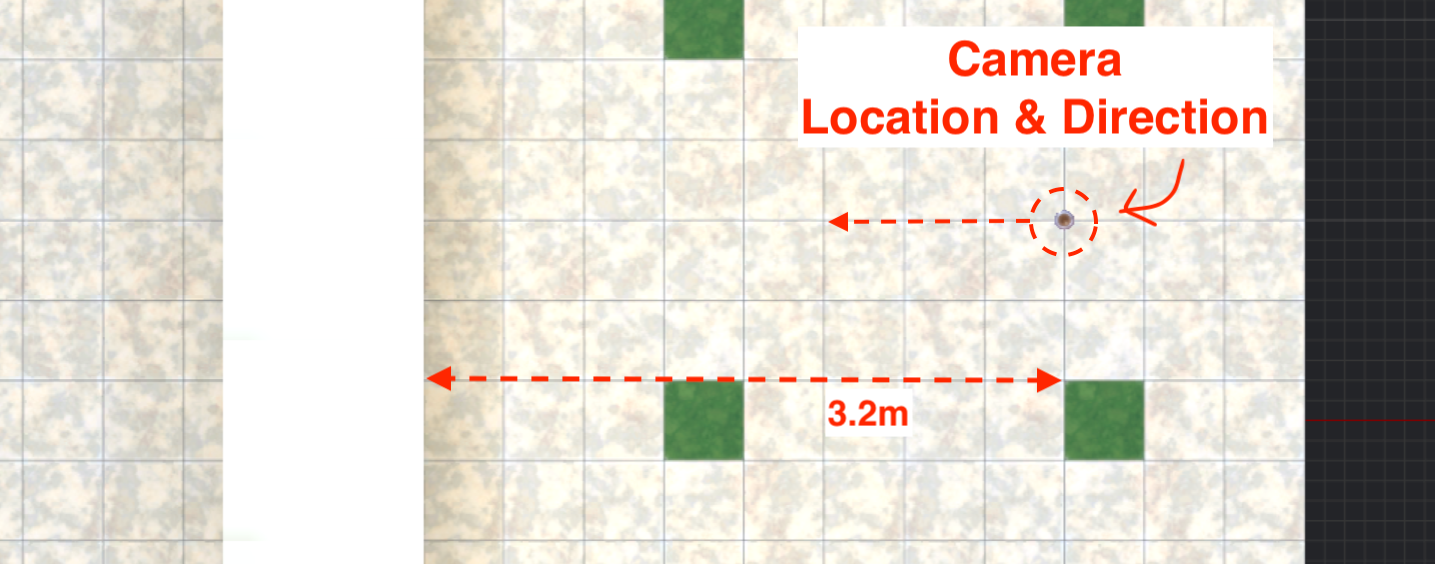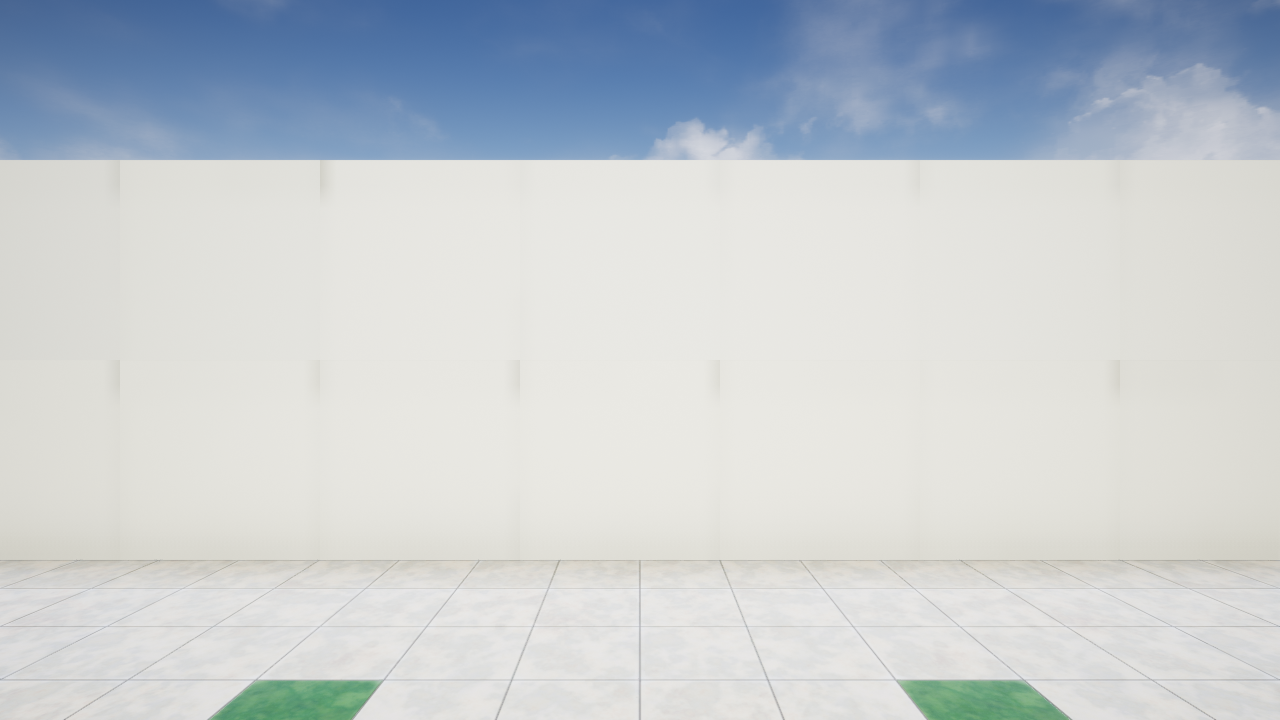Then execute the following Python script on a Jupyter Lab to get a depth of the viewport.

The colorized result looks like this. The darker pixel has a larger value (that is, further) than the lighter pixel.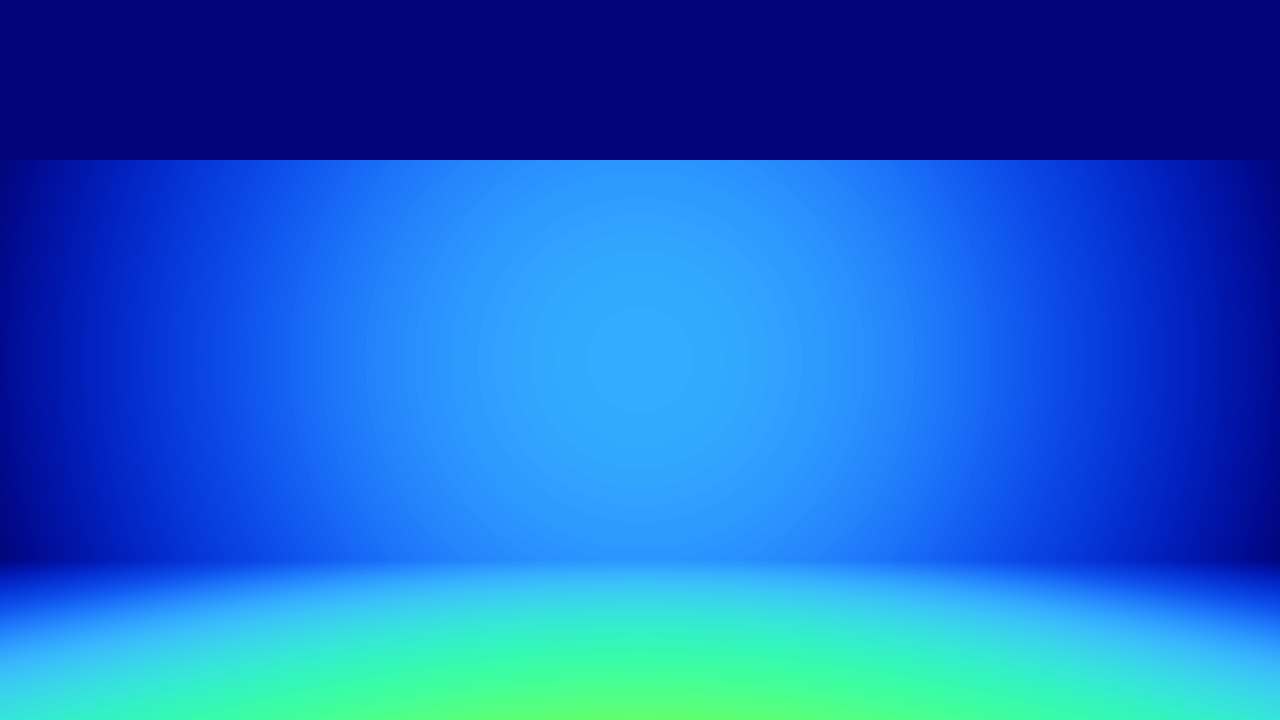If I plot the values of pixels in the horizontal centerline, the result looks like this.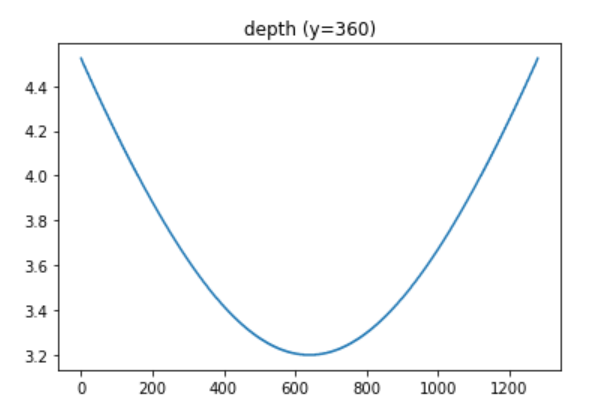I picked up two points(`[x,y]=[240,360],[480,360]`) for example, the value for each point looks like this.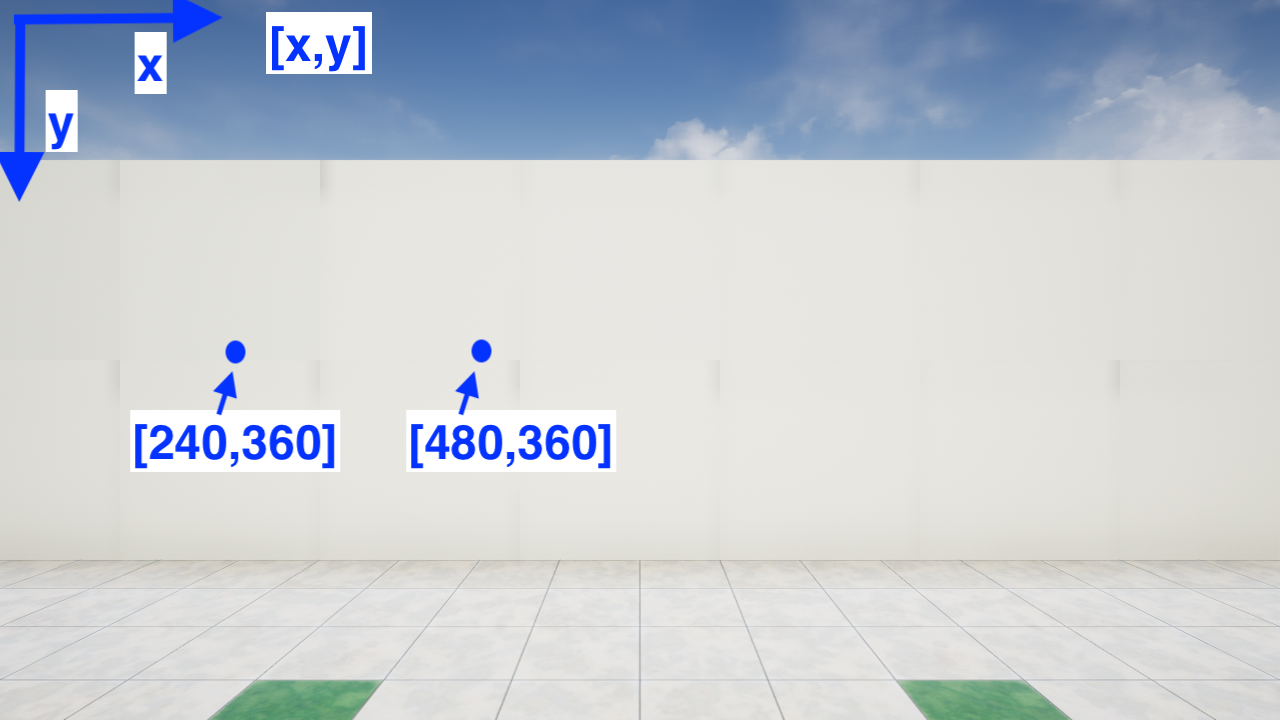``````x = 240
y = 360
print(depth[x,y]) # --> 3.7714844
x = 480
print(depth[x,y]) # --> 3.296875``````

These values(3.77 for [240,360], 3.3 for [480,360]) match the distance between the camera location and each point.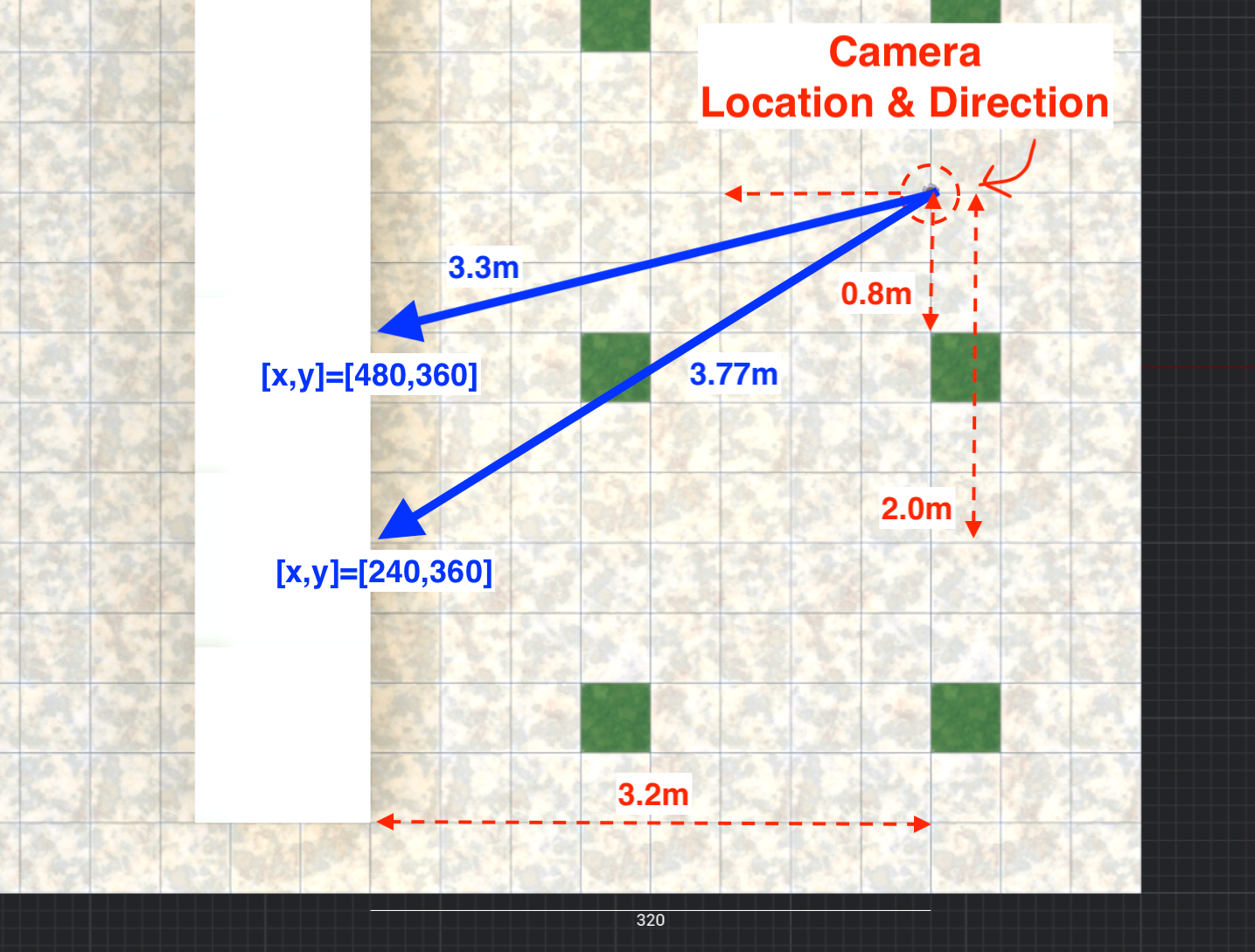## Convert Ray-Distance To Depth Map

A true depth map can be calculated with the following equations.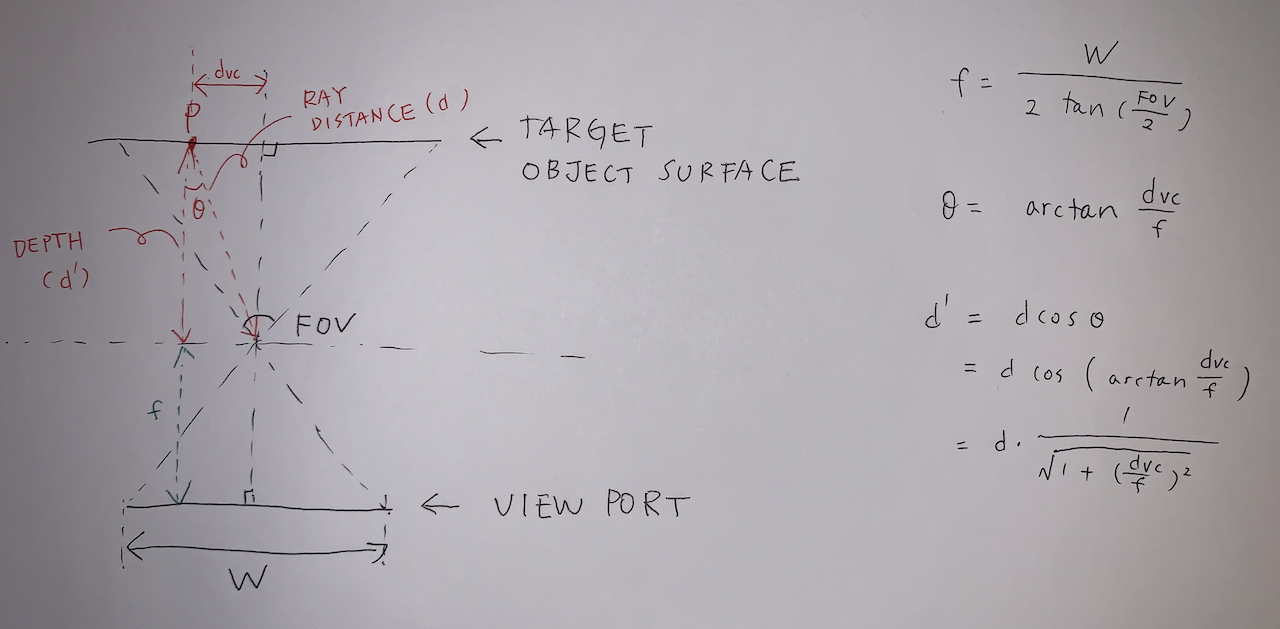The equations can be scripted like this.

The conversion result can be colorized like this.

``````FOV = 90.0
plane_depth = DepthConversion(depth, FOV)
plt.imshow(applyColorMap(plane_depth))``````If I plot the converted values of pixels in the horizontal centerline, the result looks like this(the orange one).

``````H, W = depth.shape
x = np.arange(W)
y = H / 2
plt.plot(x, depth[y,x],label='distance from camera center')
plt.plot(x, plane_depth[y,x],label='distance from camera plane')
plt.legend()
plt.title(f'depth(y={y})')
plt.show()``````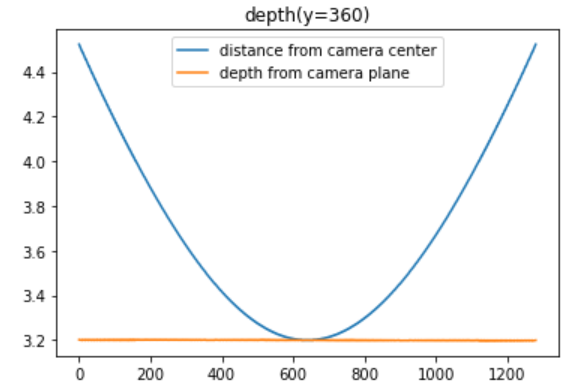## Conclusion

How to convert depth returned from UnrealCV to ordinally depth map have introduced. I heavily referenced this GitHub issue when I wrote this article. Thank you so much!

## Appendices

### Experiment Environment

#### UnrealCV Viewport Settings

• Viewport width : 1280
• Viewport height : 720
• Horizontal FOV : 90 degree

#### Software Versions

• Windows 10
• Python 3.7.7
• UnrealCV Pythonパッケージ 0.4.0 (`pip install unrealcv`)
• Unreal Engine 4.20.3
• Jupyter Lab 2.1.2

If you are unfamiliar with UnrealCV or UE4, I would recommend referring the following articles:

### Distance Measurement in UE4

In UE4, distance is represented in units called Unreal Unit(UU). 1UU is 1cm by default. This setting can be changed in the world settings.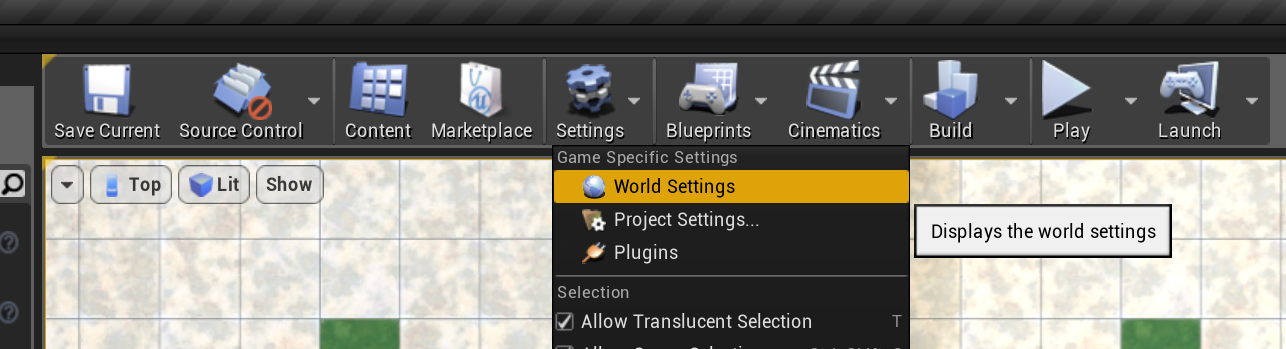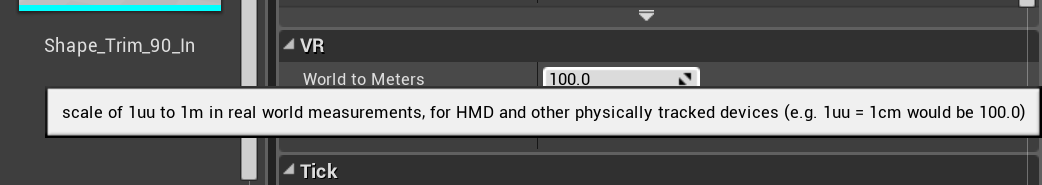You can measure a distance between two points in the UE4 level editor. First, switch the viewport to top view.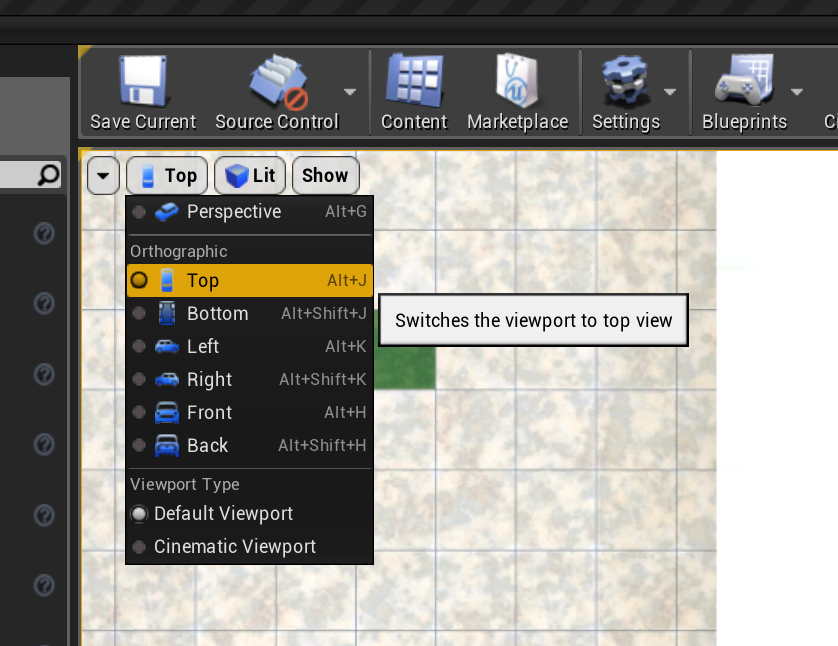Second, click the center button of your mouse at the point which you want to start the measurement, then drag your mouse cursor to the point which you want to end the measurement.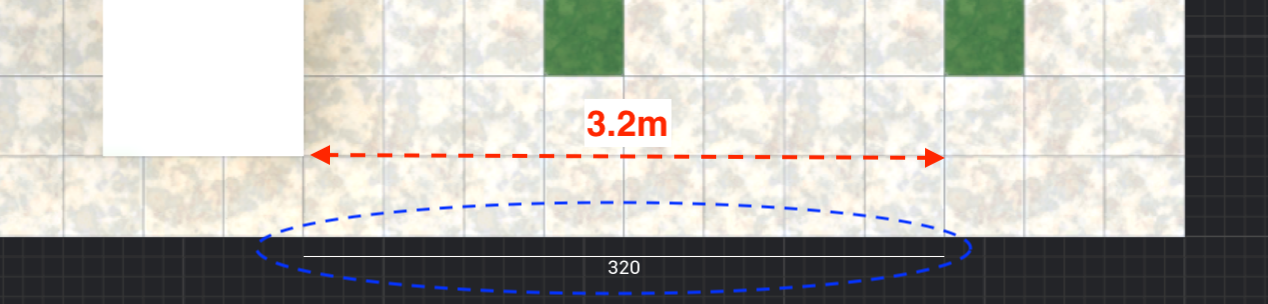## References

### Depth Map

In 3D computer graphics and computer vision, a depth map is an image or image channel that contains information relating to the distance of the surfaces of scene objects from a viewpoint.

### Colormap

I used the "Turbo" colormap which Google released in 2019 for visualizing a depth map.

Google AI Blog: Turbo, An Improved Rainbow Colormap for Visualization